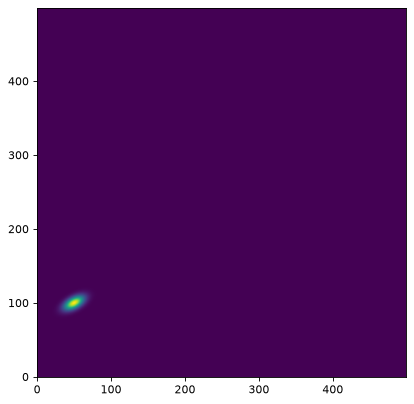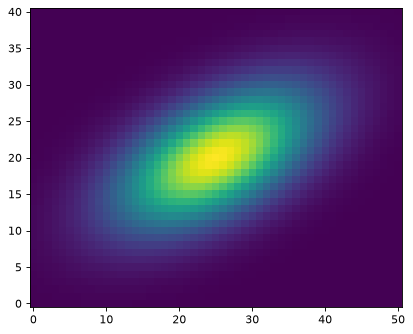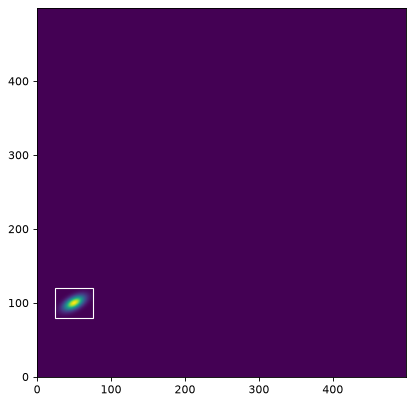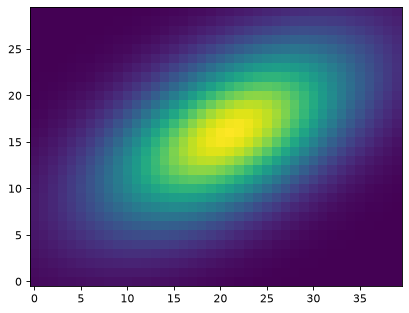# Image Utilities¶

## Overview¶

The `astropy.nddata.utils` module includes general utility functions for array operations.

## 2D Cutout Images¶

### Getting Started¶

The `Cutout2D` class can be used to create a postage stamp cutout image from a 2D array. If an optional `WCS` object is input to `Cutout2D`, then the `Cutout2D` object will contain an updated `WCS` corresponding to the cutout array.

First, we simulate a single source on a 2D data array. If you would like to simulate many sources, see Efficient Model Rendering with Bounding Boxes.

Note: The pair convention is different for size and position! The position is specified as (x,y), but the size is specified as (y,x).

```>>> import numpy as np
>>> from astropy.modeling.models import Gaussian2D
>>> y, x = np.mgrid[0:500, 0:500]
>>> data = Gaussian2D(1, 50, 100, 10, 5, theta=0.5)(x, y)
```

Now, we can display the image:

```>>> import matplotlib.pyplot as plt
>>> plt.imshow(data, origin='lower')
```

(png, svg, pdf)Next we can create a cutout for the single object in this image. We create a cutout centered at position `(x, y) = (49.7, 100.1)` with a size of `(ny, nx) = (41, 51)` pixels:

```>>> from astropy.nddata import Cutout2D
>>> from astropy import units as u
>>> position = (49.7, 100.1)
>>> size = (41, 51)     # pixels
>>> cutout = Cutout2D(data, position, size)
```

The `size` keyword can also be a `Quantity` object:

```>>> size = u.Quantity((41, 51), u.pixel)
>>> cutout = Cutout2D(data, position, size)
```

Or contain `Quantity` objects:

```>>> size = (41*u.pixel, 51*u.pixel)
>>> cutout = Cutout2D(data, position, size)
```

A square cutout image can be generated by passing an integer or a scalar `Quantity`:

```>>> size = 41
>>> cutout2 = Cutout2D(data, position, size)

>>> size = 41 * u.pixel
>>> cutout2 = Cutout2D(data, position, size)
```

The cutout array is stored in the `data` attribute of the `Cutout2D` instance. If the `copy` keyword is `False` (default), then `cutout.data` will be a view into the original `data` array. If `copy=True`, then `cutout.data` will hold a copy of the original `data`. Now we display the cutout image:

```>>> cutout = Cutout2D(data, position, (41, 51))
>>> plt.imshow(cutout.data, origin='lower')
```

(png, svg, pdf)The cutout object can plot its bounding box on the original data using the `plot_on_original()` method:

```>>> plt.imshow(data, origin='lower')
>>> cutout.plot_on_original(color='white')
```

(png, svg, pdf)Many properties of the cutout array are also stored as attributes, including:

```>>> # shape of the cutout array
>>> print(cutout.shape)
(41, 51)

>>> # rounded pixel index of the input position
>>> print(cutout.position_original)
(50, 100)

>>> # corresponding position in the cutout array
>>> print(cutout.position_cutout)
(25, 20)

>>> # (non-rounded) input position in both the original and cutout arrays
>>> print((cutout.input_position_original, cutout.input_position_cutout))
((49.7, 100.1), (24.700000000000003, 20.099999999999994))

>>> # the origin pixel in both arrays
>>> print((cutout.origin_original, cutout.origin_cutout))
((25, 80), (0, 0))

>>> # tuple of slice objects for the original array
>>> print(cutout.slices_original)
(slice(80, 121, None), slice(25, 76, None))

>>> # tuple of slice objects for the cutout array
>>> print(cutout.slices_cutout)
(slice(0, 41, None), slice(0, 51, None))
```

There are also two `Cutout2D` methods to convert pixel positions between the original and cutout arrays:

```>>> print(cutout.to_original_position((2, 1)))
(27, 81)

>>> print(cutout.to_cutout_position((27, 81)))
(2, 1)
```

### 2D Cutout Modes¶

There are three modes for creating cutout arrays: `'trim'`, `'partial'`, and `'strict'`. For the `'partial'` and `'trim'` modes, a partial overlap of the cutout array and the input `data` array is sufficient. For the `'strict'` mode, the cutout array has to be fully contained within the `data` array, otherwise an `PartialOverlapError` is raised. In all modes, non-overlapping arrays will raise a `NoOverlapError`. In `'partial'` mode, positions in the cutout array that do not overlap with the `data` array will be filled with `fill_value`. In `'trim'` mode only the overlapping elements are returned, thus the resulting cutout array may be smaller than the requested `size`.

The default uses `mode='trim'`, which can result in cutout arrays that are smaller than the requested `size`:

```>>> data2 = np.arange(20.).reshape(5, 4)
>>> cutout1 = Cutout2D(data2, (0, 0), (3, 3), mode='trim')
>>> print(cutout1.data)
[[0. 1.]
[4. 5.]]
>>> print(cutout1.shape)
(2, 2)
>>> print((cutout1.position_original, cutout1.position_cutout))
((0, 0), (0, 0))
```

With `mode='partial'`, the cutout will never be trimmed. Instead it will be filled with `fill_value` (the default is `numpy.nan`) if the cutout is not fully contained in the data array:

```>>> cutout2 = Cutout2D(data2, (0, 0), (3, 3), mode='partial')
>>> print(cutout2.data)
[[nan nan nan]
[nan  0.  1.]
[nan  4.  5.]]
```

Note that for the `'partial'` mode, the positions (and several other attributes) are calculated for on the valid (non-filled) cutout values:

```>>> print((cutout2.position_original, cutout2.position_cutout))
((0, 0), (1, 1))
>>> print((cutout2.origin_original, cutout2.origin_cutout))
((0, 0), (1, 1))
>>> print(cutout2.slices_original)
(slice(0, 2, None), slice(0, 2, None))
>>> print(cutout2.slices_cutout)
(slice(1, 3, None), slice(1, 3, None))
```

Using `mode='strict'` will raise an exception if the cutout is not fully contained in the data array:

```>>> cutout3 = Cutout2D(data2, (0, 0), (3, 3), mode='strict')
PartialOverlapError: Arrays overlap only partially.
```

### 2D Cutout from a `SkyCoord` Position¶

The input `position` can also be specified as a `SkyCoord`, in which case a `WCS` object must be input via the `wcs` keyword.

First, we define a `SkyCoord` position and a `WCS` object for our data (usually this would come from your FITS header):

```>>> from astropy.coordinates import SkyCoord
>>> from astropy.wcs import WCS
>>> position = SkyCoord('13h11m29.96s -01d19m18.7s', frame='icrs')
>>> wcs = WCS(naxis=2)
>>> rho = np.pi / 3.
>>> scale = 0.05 / 3600.
>>> wcs.wcs.cd = [[scale*np.cos(rho), -scale*np.sin(rho)],
...               [scale*np.sin(rho), scale*np.cos(rho)]]
>>> wcs.wcs.ctype = ['RA---TAN', 'DEC--TAN']
>>> wcs.wcs.crval = [position.ra.to_value(u.deg),
...                  position.dec.to_value(u.deg)]
>>> wcs.wcs.crpix = [50, 100]
```

Now we can create the cutout array using the `SkyCoord` position and `wcs` object:

```>>> cutout = Cutout2D(data, position, (30, 40), wcs=wcs)
>>> plt.imshow(cutout.data, origin='lower')
```

(png, svg, pdf)The `wcs` attribute of the `Cutout2D` object now contains the propagated `WCS` for the cutout array. Now we can find the sky coordinates for a given pixel in the cutout array. Note that we need to use the `cutout.wcs` object for the cutout positions:

```>>> from astropy.wcs.utils import pixel_to_skycoord
>>> x_cutout, y_cutout = (5, 10)
>>> pixel_to_skycoord(x_cutout, y_cutout, cutout.wcs)
<SkyCoord (ICRS): (ra, dec) in deg
( 197.8747893, -1.32207626)>
```

We now find the corresponding pixel in the original `data` array and its sky coordinates:

```>>> x_data, y_data = cutout.to_original_position((x_cutout, y_cutout))
>>> pixel_to_skycoord(x_data, y_data, wcs)
<SkyCoord (ICRS): (ra, dec) in deg
( 197.8747893, -1.32207626)>
```

As expected, the sky coordinates in the original `data` and the cutout array agree.

### 2D Cutout Using an Angular `size`¶

The input `size` can also be specified as a `Quantity` in angular units (e.g., degrees, arcminutes, arcseconds, etc.). For this case, a `WCS` object must be input via the `wcs` keyword.

For this example, we will use the data, `SkyCoord` position, and `wcs` object from above to create a cutout with size 1.5 x 2.5 arcseconds:

```>>> size = u.Quantity((1.5, 2.5), u.arcsec)
>>> cutout = Cutout2D(data, position, size, wcs=wcs)
>>> plt.imshow(cutout.data, origin='lower')
```

(png, svg, pdf)## Saving a 2D Cutout to a FITS File with an Updated WCS¶

A `Cutout2D` object can be saved to a FITS file, including the updated WCS object for the cutout region. In this example, we download an example FITS image and create a cutout image. The resulting `Cutout2D` object is then saved to a new FITS file with the updated WCS for the cutout region.

```# Download an example FITS file, create a 2D cutout, and save it to a
# new FITS file, including the updated cutout WCS.
from astropy.io import fits
from astropy.nddata import Cutout2D
from astropy.wcs import WCS

# Load the image and the WCS
hdu = fits.open(filename)

# Make the cutout, including the WCS
cutout = Cutout2D(hdu.data, position=position, size=size, wcs=wcs)

# Put the cutout image in the FITS HDU
hdu.data = cutout.data

# Update the FITS header with the cutout WCS